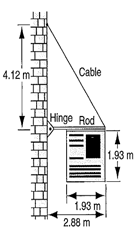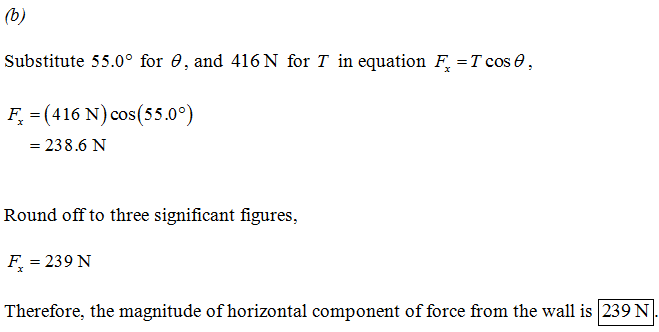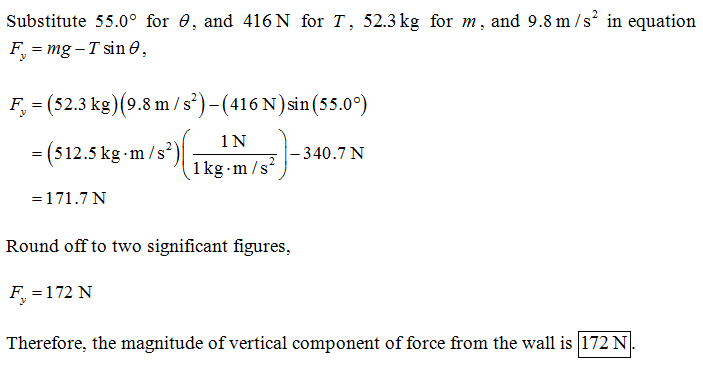×#### Thank you for registering.

One of our academic counsellors will contact you within 1 working day.

Click to Chat

1800-1023-196

+91-120-4616500

CART 0

• 0

MY CART (5)

Use Coupon: CART20 and get 20% off on all online Study Material

ITEM
DETAILS
MRP
DISCOUNT
FINAL PRICE
Total Price: Rs.

There are no items in this cart.
Continue Shopping
```
A 52.3-kg uniform square sign, 1.93 m on a side, is hung from a 2.88-m rod of negligible mass. A cable is attached to the end of the rod and to a point on the wall 4.12 m above the point where the rod is fixed to the wall, as shown in Fig. (a) Find the tension in the cable. (b) Calculate the horizontal and vertical components of the force exerted by the wall on the rod.
A 52.3-kg uniform square sign, 1.93 m on a side, is hung from a 2.88-m rod of negligible mass. A cable is attached to the end of the rod and to a point on the wall 4.12 m above the point where the rod is fixed to the wall, as shown in Fig. (a) Find the tension in the cable. (b) Calculate the horizontal and vertical components of the force exerted by the wall on the rod.```
5 years ago

``````
5 years ago
```							(a) Assume that each of the two support points for the square sign experience the same tension, equal to half of the weight of the sign. The net torque on the rod about an axis through the hinge is (52.3kg/2)(9.81m/s2)(0.95m)+(52.3kg/2)(9.81m/s2)(2.88m)−(2.88m)T sinθ = 0, where T is the tension in the cable and θ is the angle between the cable and the rod. The angle can be found from θ = arctan(4.12m/2.88m) = 55.0◦, so T = 416N. (b) There are two components to the tension, one which is vertical, (416N)sin(55.0◦) = 341N, and another which is horizontal, (416N)cos(55.0◦) = 239N. The horizontal force exerted by the wall must then be 239N. The net vertical force on the rod is F +(341N)−(52.3kg/2)(9.81m/s2) = 0, which has solution F = 172N as the vertical upward force of the wall on the rod.
```
5 days ago
Think You Can Provide A Better Answer ?

## Other Related Questions on Mechanics

View all Questions »### Course Features

• 101 Video Lectures
• Revision Notes
• Previous Year Papers
• Mind Map
• Study Planner
• NCERT Solutions
• Discussion Forum
• Test paper with Video Solution### Course Features

• 110 Video Lectures
• Revision Notes
• Test paper with Video Solution
• Mind Map
• Study Planner
• NCERT Solutions
• Discussion Forum
• Previous Year Exam Questions